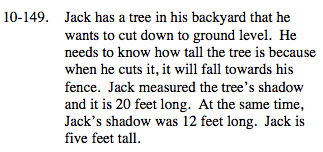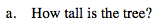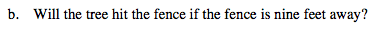### Home > MC2 > Chapter 10 > Lesson 10.2.6 > Problem10-149

10-149.Since the shadow lengths are proportional to their heights, we can create an equation using the given ratios.

Relate the shadow length to height ratios of the tree and Jack to solve for the unknown variable of the tree's height.

$\frac{20}{\it x} = \frac{12}{5}$

Tree's height = 8.33 ft.Based on the answer from part (a), was the tree's height taller than nine feet?